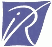#Robert Harley

At the 1993 conference, John Conway and Andrew Odlyzko defined a "high-jumper" as a number which occurs most frequently as the difference of consective primes <= x for some x and conjectured that the only high-jumpers are 4 and the prime factorials 2, 6, 30, 210, 2310... Victor Meally asked when 30 takes over from 6 as the commonest gap.

To answer that question, I computed values of Brent's T_{r,k} function, hundreds of digits of the twin prime constant and other Hardy-Littlewood constants and various logarithmic integrals to use in a calculation, based on the prime k-tuples conjecture, for estimating the crossover point (it's somewhere around 174274357323366788616982464713243872 i.e., about 1.7×10^35).

You can download the paper about this as a 7K .dvi.gz file and an accompanying 2K .ps.gz figure, or the two of them in a 266K .ps file. Here are the first few Hardy-Littlewood constants to 45 digits:

c_2 = 0.66016 18158 46869 57392 78121 10014 55577 84326 23360+
c_3 = 0.63516 63546 04271 20720 66965 91272 52241 73420 65687+
c_4 = 0.30749 48787 58327 09312 33544 86071 07685 30221 78520-
c_5 = 0.40987 48850 88236 47447 87812 12337 95527 78963 58013+
c_6 = 0.18661 42973 58358 39665 69248 47944 18833 78400 73945-
c_7 = 0.36943 75103 86498 68932 31907 49876 75077 70553 72914-
c_8 = 0.23241 93345 86716 54620 61302 12670 66423 16017 58033-
c_9 = 0.12017 12067 74741 72146 99894 64638 47738 34654 94083-
c_10 = 0.04180 40508 12181 65710 39748 72842 46412 05882 81078+
c_11 = 0.09453 08285 13547 15843 37971 55991 07208 84456 47523+
c_12 = 0.03539 32598 44463 70442 74555 96801 94105 85290 48911-
c_13 = 0.11170 39095 63244 95377 12104 54656 08232 51503 03622+
c_14 = 0.06284 46339 43607 42176 34598 50911 39350 53388 62666+
c_15 = 0.02924 41621 25091 30867 18534 29424 46031 81891 89082-
c_16 = 0.00922 81011 53092 67664 26576 54374 72155 09035 30929+

A table of the T_{r,k} function is here. I would like to find out where 210 overtakes 30 but that would require computing T_{r, k} for r = 105...INRIA, Domaine de Voluceau - Rocquencourt, 78153 Le Chesnay, France.

Please note: These pages are no longer maintained (since circa December 2000).